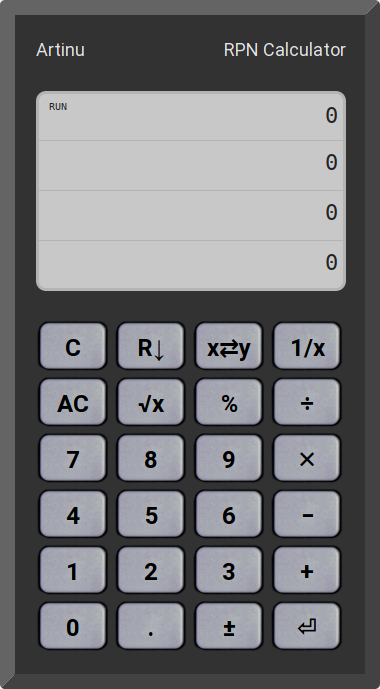# Image Recognition and Linear Regression

In the following article, we will look at image recognition using linear regression. We realize that this idea, at first glance, may seem quite unusual. However, we will show using a simple example, that for a certain class of images, and under quite strictly defined circumstances, the linear regression method can achieve surprisingly fair results.

# RPN Calculator

Reverse Polish Notation is a method of notation of mathematical expressions that allows simple calculations to be performed without the need of using brackets – thanks to the use of a stack. This method has been popularized by Hewlett Packard, which has been successfully using it in its calculators for many years.

The Artinu application is a simple RPN calculator, written in JavaScript, available online:# COVID-19 Death Rate

Update:

We present death rate versus time chart for selected countries: Brazil, Germany, Italy, US, Poland, Sweden, Switzerland. Death rate is calculated as follows:
$$d = \frac{N_\mathrm{deaths}}{N_{\mathrm{infected}}} \cdot 100,$$
where $$N_\mathrm{deaths}$$ is number of deaths caused by COVID-19 infection since the beginning of epidemic, $$N_{\mathrm{infected}}$$ is a total number of infected people. For each country, the plot starts at the first date where there are at least 100 infected cases, which is common practice.

# Biased and unbiased estimators

When we want to know a standard deviation of a big population, we usually take a sample from the whole and than calculate estimator value. However it is not always clear which estimator should we use. Sometimes people argue whenever biased or unbiased standard deviation estimator is better. Below we explore this field and present the result of the numerical simulation.

# Czy sztuczna inteligencja zna się na mechanice kwantowej?

We explore the possibility of the K-Means algorithm usage for cleaning scans of hand-made notes. A Scikit Learn implementation of the algorithm is used. The original image is decomposed into three clusters in RGB space. Finally we got cleaned picture as the result of removing 2 of 3 clusters from the original one. References:

Jakiś czas temu, podczas porządkowania szafy wpadły mi w ręce, moje stare szpargały. Notatki z wykładów z mechaniki kwantowej, które to notatki jako student w latach 90-tych skrzętnie prowadziłem. Gdy już się nacieszyłem wspomnieniami zacząłem się zastanawiać czy nie dałoby się nieco poprawić ich wyglądu, oczyścić ze zbędnych elementów. Na każdej stronie widnieje niebiesko-blada kratka, dodatkowo pojawiają się przebitki atramentu z drugiej strony kartki. Widoczne są również otwory na wpięcie do segregatora.

# The limits of central limit theorem – part 2

In the previous part we made look through the distribution of sample means for three distributions: Uniform, Cauchy, and Petersburg distribution. The Cauchy and Petersburg distributions do not fulfill the Central Limit Theorem since they have infinite variance (and infinite expected value in “Petersburg” case). Now we will have a look at the numerical results for standard deviation of sample means. As in previous part, we use Uniform distribution only as a reference since it fulfills CLT and we use the same pseud-random number generator (Mersenne-Twister).

# The limits of central limit theorem

The power of Central Limit Theorem is widely known. In the following post we are exploring a bit the areas outside its scope – where the CLT does not work. We present the results of numerical simulations for three distributions: Uniform, Cauchy distribution, and certain “naughty” distribution called later “Petersburg distribution”.